×#### Thank you for registering.

One of our academic counsellors will contact you within 1 working day.

Click to Chat

1800-1023-196

+91-120-4616500

CART 0

• 0

MY CART (5)

Use Coupon: CART20 and get 20% off on all online Study Material

ITEM
DETAILS
MRP
DISCOUNT
FINAL PRICE
Total Price: Rs.

There are no items in this cart.
Continue Shopping• Complete Physics Course - Class 11
• OFFERED PRICE: Rs. 2,968
• View Details

```Kirchhoff’s Law

Current and Voltage

Kirchhoff’s Laws

Circuit terms

The First Law - Kirchhoff’s Current Law

The Second law – Kirchhoff’s Voltage Law

Circuit Analysis

Applications of Kirchhoff’s Law

Summary

Current and Voltage

Before moving into Kirchhoff’s Laws let us discuss about the current and voltage in a network as the law deals with both voltage and current.

In metals, the electrons in the outermost orbit are not tightly bonded and so these electrons leave those respective atom and float around. These electrons are termed as free electrons. Thus the electrical conductivity is the flow of these free electrons. Conductors such as copper, silver allow the free movement of outermost electrons from one atom to another. Insulators like wood and rubber does not allow the movement of electrons between the atoms as they are held tightly within the atoms. The direction of electric current will be opposite to the direction of flow of electrons.

Electric current is defined as the rate at which an electrical charge passes through a point. It is the flow of positive charges. An electric current of one ampere flows through the material, if one coulomb of charge passes through the conductor in one second. The SI unit of current is amperes. The higher the rate at which electrons flow in a circuit, the higher is the flow of current.

Electric current flows due to the difference in potential between two points. Potential difference is the variation in the number of negative charges that causes the flow of electrons. The negative potential is the point with more electrons and the positive potential is the point with less number of electrons. So electrons flow from negative potential to positive potential. Thus the current flows from positive potential end to the negative potential end when there will be potential difference. This potential difference is mentioned as the voltage in a circuit. Voltage is defined as the energy required to move a unit charge from one point to another. The unit of voltage is volt. One joule per coulomb is called a volt.

The amount of current in a circuit is determined by the difference in voltage. For Example when no current passes through a bulb, it means that the voltage at both sides of the bulb is the same. The potential difference is usually provided by batteries.  Consider some light bulbs which are connected to a battery in parallel. The voltage across each bulb will be equal to the voltage produced by the battery. In this case the total current which is taken from the battery is equal to the sum of all currents which is drawn by each bulbs. When the light bulbs are connected in series the current that passes through each bulb is the same and thus the light will not be as bright.

Gustav Robert Kirchhoff

Gustav Robert Kirchhoff was a scientist in the nineteenth century who contributed very much for the circuit theory. Thus he could provide a better understanding about the electrical circuits. He discovered that through a conductor the electric current flows at the speed of light.

Kirchhoff’s Laws

Gustav Kirchhoff developed a set of laws relating to the conservation of current and energy in the electrical circuits. They are KCL (Kirchhoff’s Current Law) which deals with the current flowing in the circuit and KVL (Kirchhoff’s Voltage Law) which deals with the voltage source present in the circuit. His discoveries has also paved the path for quantum theory of electromagnetic induction by Max Planck. Most of his discoveries and researches were dealing with electric current. Among this Kirchhoff’s law of circuits is the most important one.

We know that Georg Simon ohm showed the relationship between voltage, current and resistance and formulated the ohm’s law. This law is the basis of electricity. The law states that V = I R, where voltage V is in volts, Current I in amps and resistance R in ohms. Thus I = V / R and R = V / I. But in complex circuits it is difficult to find the voltage and current in the circuit by using Ohm’s law. Hence for complex circuits Kirchhoff’s law of circuits help us to find the value of voltage and current which flows within the circuit.

Circuit terms

A circuit is a closed path through which current flows. A path is a single line which consists of circuit elements and sources. A node is defined as a terminal or junction in which two or more elements will be joined together and thus have a common point for more than one branches. A branch consists of elements like resistors and sources connected between two nodes. A loop is a closed path in which elements are counted just once. A mesh is an open loop and open path with no elements in it. If the elements are connected in series the current which flows through each ones are the same. If the elements are connected in parallel the voltage across each components remains the same.

The First Law - Kirchhoff’s Current Law

This law deals with the current flowing in the circuit. It states that the algebraic sum of all the currents meeting at a point is zero. The total current entering a node or junction is equal to the total current or charge leaving the node. This is also known as the Conservation of Charge.

Consider a node and here three currents are entering the node and three currents are leaving the node. The currents entering the node are taken as positive and the current leaving the node is taken as negative. The law states that the totals current entering the node plus the total currents leaving the node is equal to zero.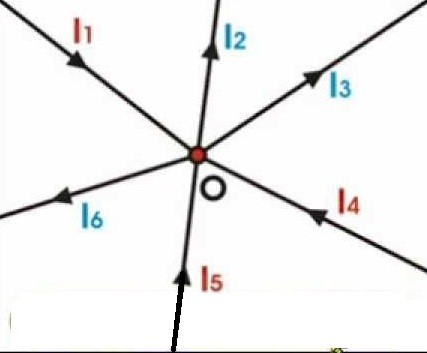Kirchhoff’s current law

Thus I1 – I2 - I3 + I4 + I5 – I6 = 0 or I1 + I4 + I5 = I2 + I3 + I6. This is the junction rule.

So currents entering to a node = currents leaving the node.

The Second Law – Kirchhoff’s Voltage Law

When current travels in a circuit the magnitude of the current changes according to the product of current and resistance or emf which is connected in the circuit. Thus the law states that the algebraic sum of all voltages within the loop will be equal to zero. This is also known as the Conservation of Energy. Here the sum of voltage drops is equal to the sum of voltage rise. Consider three voltages V1, V2, V3  which is connected in a circuit.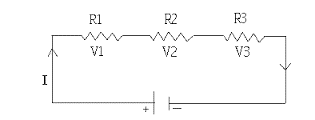Kirchhoff’s voltage law

The Equation according to the law is written as V1 + V2 + V3 = 0.

The direction of the current can be either taken in clockwise direction or in anticlock wise direction. Once selecting the direction of the current we need to maintain the same direction throughout the circuit. If the final value is positive then it is clear that the direction of current assumed is right. If the final value we get is a negative value then it says that the current direction we assumed is reversed. The voltage drops across the resistors when the current flows in clock wise n clockwise direction is considered as positive drops. The voltage drops across the resistors when the current flows in anticlock wise direction is taken as negative drops. This is also known as the Loop Rule.

Circuit Analysis

Here we are solving the circuit given below by using Kirchhoff’s current law and Kirchhoff’s voltage law. Consider a circuit by resistors R1, R2 and R3  whose values are 5 Ω, 10 Ω and 5 Ω respectively. Two cells E1 and E2 are connected in the circuits whose values are 10 V and 5 V respectively.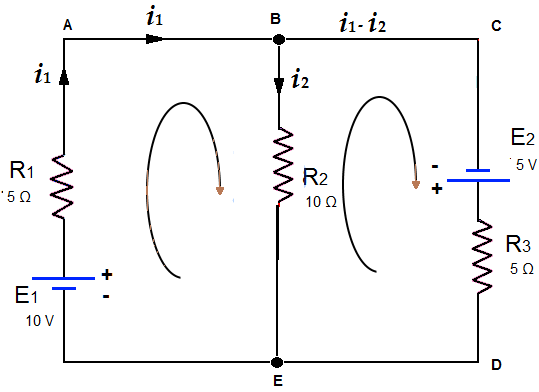Solving the circuit by KCL and KVL

Consider the direction of current flow as indicated by arrows within the circuit. Now let us apply Kirchhoff’s current law to the junctions B and E.

So current in the mesh EAB = i1.

Current in the mesh BE = i2.

Thus current in the mesh BCDE = i1 – i2.

Next we are applying Kirchhoff’s voltage law on the mesh EAB.  Here 10 V is considered in the clock wise direction.

5 i1 + 10 i2 = 10. Let it be the first equation.

Consider the next mesh EBCD in which 5 V is again in the clock wise direction.

5 (i1 – i2) – 10 i2 = 5

5 i1 – 5 i2 – 10 i2 = 5

5 i1 - 15 i2 = 5

Solving the two equations we get

5 i1 + 10 i2 = 10 –

5 i1 - 15 i2 = 5

Then 10 i2 – (- 15i2) = 5

10 i2 + 15 i2  = 5

Thus 25 i2 = 5

i2 = 5/25 = 0.2 A

Substitute this value in equation 1 we get   5 i1 + 10 (0.2) = 10

5 i1 + 2 = 10

5 i1 = 8

i1 = 8 / 5 = 1.6 A

Thus i1 = 1. 6 A and i2 = 0.2 A

1. 6 A is the current that flows through the mesh EAB of 5 Ω resistance. 0.2 A is the current that flows through 10 Ω

i1 - i2 = 1.6 - 0.2 = 1.4 Amp is the current that flows in the mesh BCDE with the resistance 5 Ω.

Finding the internal resistance and emf of cells by Kirchhoff’s law

EMF or electromotive force is the potential difference that is developed between the two terminals of a battery in an open circuit. The internal resistance is the resistance which is provided by the electrolyte and electrodes that is present in a cell. So Internal resistance is offered by the electrodes and electrolyte which oppose the current flow inside the cell.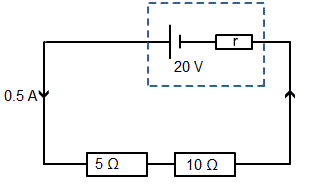To find the internal resistance

We know that the total amount of energy within the circuit is equal to the total amount of energy taken out. So energy in = energy out. We also know that according to ohm’s law V = I R, where I is the current and R is the resistance.

20 V = (0.5 * 5) + (0.5 * 10) + (0.5 * r)

= 2.5 + 5 + 0.5 r

= 7.5 + 0.5 r

20 – 7.5 = 0.5 r

0.5 r = 12.5

r = 12.5 / 0.5

= 25 Ω

Thus we could find the internal resistance of a cell.

Next we are going to find the emf of the circuit given below. From the second law we can write the equation as 1.5 * 5 + 1.5 * 2 + 1.5 * 3 = emf

Thus emf = 7.5 + 3 + 4.5

= 15 V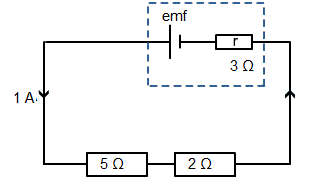To find the emf

The emf of a battery that causes the current to flow in clock wise direction will be positive and the emf of a battery which makes the current to flow in anticlock wise direction is negative.

Applications of Kirchhoff’s Law

We understood that Kirchhoff’s law is used to find the values of current voltage and internal resistance in DC circuits. Thus we are able to find out the unknown values in complex networks and circuits. Wheatstone bridge is an important application of Kirchhoff’s law. It is used in mesh and node analysis.

Limitations of Kirchhoff’s law

Both the law KCL and KVL are not suitable for AC circuits of high frequencies. Current laws applicable only when the electric charge in a circuit is constant. KVL is applied in an assumption that magnetic fields do not change in a closed circuit. So we cannot apply KVL when magnetic field varies within a circuit.

Summary

Gustav Kirchhoff provided a better understanding about the electrical circuits.

The first law of Kirchhoff states that the total current that enters a node or junction is equal to the total current or charge leaving the node. It is known as the Conservation of Charge.This is the junction rule.

The second law of Kirchhoff states that the sum of voltage drops is equal to the sum of voltage rise. It is known as the Conservation of Energy. This is the loop rule.

Watch this Video for more reference

Kirchhoff’s Law
```### Course Features

• 101 Video Lectures
• Revision Notes
• Previous Year Papers
• Mind Map
• Study Planner
• NCERT Solutions
• Discussion Forum
• Test paper with Video Solution